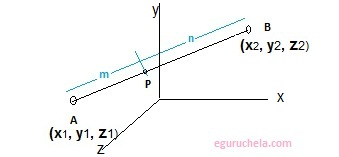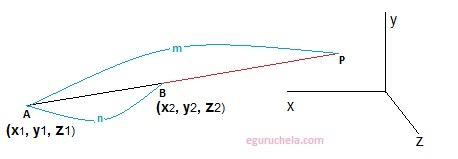Coordinates of Points Externally/Internally(3 dimension) Calculator

 X1: Y1: Z1: X2: Y2: Z2: Ratio m: n:

 Externally Internally

 Coordinates of Point

Calculator find the coordinates of point p which divides the line joining two Entered points A and B internally or externally, in a specified ratio m and n. Coordinates of point is a set of values that is used to determine the position of a point in a three dimensional Cartesian coordinate.

Internally divided line segment:

The given three dimensional coordinates forms a line AB where point P(xp, yp, yp) lies outside of the line segment AB. It can divide the AB externally as showing below:The formula used for internally division of a line segment as follows:

$$( \frac {mx_{\,2} + nx_{\,1} }{m + n},\frac {my_{\,2} + ny_{\,1} }{m + n} ,\frac {mz_{\,2} + nz_{\,1} }{m + n} )$$

Externally divided line segment:

The given 3D coordinates forms a line AB where point P(xp, yp, zp) lies on the line segment AB joining Point P. It is showing bellow:The formula used for externally division of a line segment as follows:

$$( \frac {mx_{\,2} - nx_{\,1} }{m - n},\frac {my_{\,2} - ny_{\,1} }{m - n} ,\frac {mz_{\,2} - nz_{\,1} }{m - n} )$$